Multiplying
FOIL Method

One method that can be used when multiplying two binomials is the FOIL method. FOIL stands for First, Outer, Inner, and Last. These letters help to remember to multiply each term in the first binomial by each term in the second binomial using the Distributive Property.

Example Given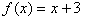and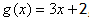, find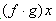.

Step 1. Replace f(x) and g(x) with their equivalent expressions.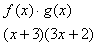Step 2. Multiply the First, Outer, Inner, and Last terms of each binomial.

First + Outer + Inner + Last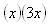+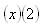+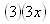+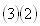Step 3. Simplify the expressions.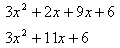For a review of the FOIL method of multiplying, please select the following link:

Listen